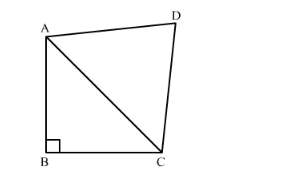# In a quadrilateral ABCD, ∠B = 90°, AD2 = AB2 + BC2 + CD2,

Question:

In a quadrilateral $A B C D, \angle B=90^{\circ}, A D^{2}=A B^{2}+B C^{2}+C D^{2}$, prove that $\angle A C D=90^{\circ}$.

Solution:In order to prove angle $\angle A C D=90^{\circ}$ it is enough to prove that $A D^{2}=A C^{2}+C D^{2}$.

Given, $A D^{2}=A B^{2}+B C^{2}+C D^{2}$

$A D^{2}-C D^{2}=A B^{2}+B C^{2}$.....(1)

Since $\angle B=90^{\circ}$, so applying Pythagoras theorem in the right angled triangle $\mathrm{ABC}$, we get

$A C^{2}=A B^{2}+B C^{2}$......(2)

From (1) and (2), we get

$A C^{2}=A D^{2}-C D^{2}$

$A C^{2}+C D^{2}=A D^{2}$

Therefore, angle $\angle A C D=90^{\circ}$ (Converse of pythagoras theorem)